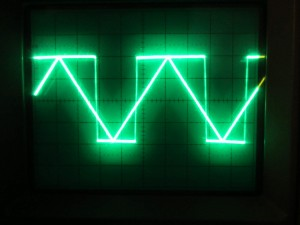Hysteresis can be added to a comparator circuit to improve its stability, especially when the input signal is noisy. In this post, we will examine the hysteresis characteristics of some common comparator and Op Amps using an oscilloscope.

Perhaps the most intuitive way to visualize the hysteresis in a circuit is to plot the input signal (x axis) against the output signal (y axis). So, if we sweep the input voltage we should be able to see the characteristics of the transitioning of the output voltage due to hysteresis.

The test circuit I am going to use is as follows: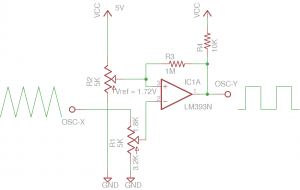R1 (potentiometer) and R3 determine the trip voltage and hysteresis. Potentiometer R2 serves as a crude voltage reference and sets the reference voltage at the inverting input. R4 is a pull up resistor for comparators with open-collector outputs.

Here is a picture of the setup on breadboard. The potentiometer on the left (which corresponds to R1) sets the reference voltage at 1.72V. This value is used for all the tests conducted below. The actual voltage set for the reference is not very important however, it was mainly chosen for easy visualization and comparison purpose. The potentiometer (R1) connected to the negative input was adjusted to be 1.8K and 3.2K (from input to wiper, and wiper to ground). Again, the wiper position is fixed for all the tests.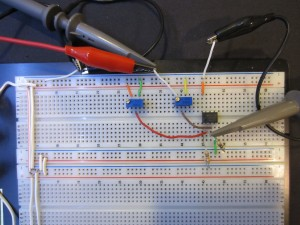Because a comparator’s hysteresis depends on many different factors such as the output voltage swing and input bias current, etc., you will see from the pictures below that even with the other settings unchanged (i.e. same resistor values), the hysteresis changes significantly depending on the comparator/op amps used. So to calculate hysteresis accurately, the characteristics of the comparator/op amp must be precisely known.

This app note from Maxim Integrated gives some good examples on how to calculate hysteresis using comparators with either open-collector output or push-pull output.

For our experiments, the supply voltage is set to 5V. And a 1 KHz 0-5V triangular waveform is used for the input sweep. The oscilloscope is set to X-Y mode with X axis tied to the triangular waveform (also the input into the Op Amp via R1) and the Y axis is connected to the Op Amp output (pin 1). The X axis is set to 0.1V/div and the Y axis is set to 1V/div.

Here is a picture showing the hysteresis when using an LM393 comparator. Using the component values mentioned earlier, we have a 50mV hysteresis.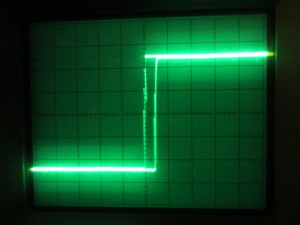The picture below shows the hysteresis characteristics of NE5532, a fast Op Amp. With everything else remains the same, the hysteresis measured here is around 80mV.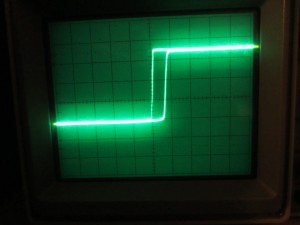Generally speaking, we should use dedicated comparators for this kind of open-looped circuits (see Analog Devices‘ application notes here and here) except perhaps in situations where lowering input bias current and input offset voltage is important. In general, comparators have much faster transition characteristics compared to Op Amps as they generally do not have the compensation capacitor built in and thus have a much higher slew rate. As you can see, even though NE5532 is a fast Op Amp it still has noticeable slower transitions compared to LM393.

The picture below shows the output using another popular Op Amp (LM358). Because of its low gain-bandwidth product), the effect of the low slew rate is clearly visible. The hysteresis exhibited here is also much larger at around 0.5V.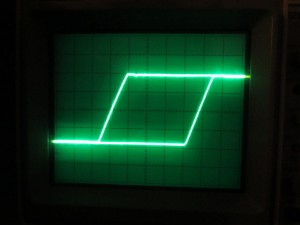In this picture, we take a look another general purpose Op Amp LMC662. LMC662 has extremely low input bias current (~fA). While its output is rail-to-rail, its input clearly is not and this is apparent from the distorted output signal. The hysteresis is at around 0.8V.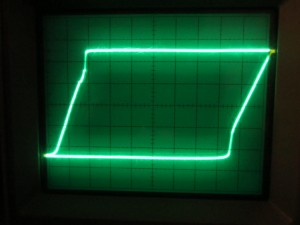The hysteresis can also be observed in time domain. The following picture shows the input and output waveforms superimposed (LM358), and the hysteresis is the diference between the two points where the rising edge and falling edge of the square wave intercept with the input triangular wave.